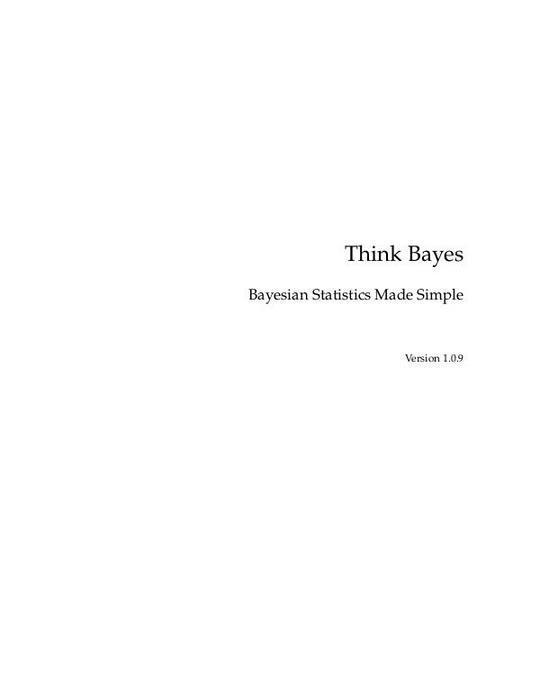Free

# thinkbayes

By Unknown
Free
The publisher has enabled DRM protection, which means that you need to use the BookFusion iOS, Android or Web app to read this eBook. This eBook cannot be used outside of the BookFusion platform.
Book Description
• Preface
• My theory, which is mine
• Modeling and approximation
• Working with the code
• Code style
• Prerequisites
• Bayes's Theorem
• Conditional probability
• Conjoint probability
• The cookie problem
• Bayes's theorem
• The diachronic interpretation
• The M&M problem
• The Monty Hall problem
• Discussion
• Computational Statistics
• Distributions
• The cookie problem
• The Bayesian framework
• The Monty Hall problem
• Encapsulating the framework
• The M&M problem
• Discussion
• Exercises
• Estimation
• The dice problem
• The locomotive problem
• What about that prior?
• An alternative prior
• Credible intervals
• Cumulative distribution functions
• The German tank problem
• Discussion
• Exercises
• More Estimation
• The Euro problem
• Summarizing the posterior
• Swamping the priors
• Optimization
• The beta distribution
• Discussion
• Exercises
• Odds and Addends
• Odds
• The odds form of Bayes's theorem
• Oliver's blood
• Maxima
• Mixtures
• Discussion
• Decision Analysis
• The Price is Right problem
• The prior
• Probability density functions
• Representing PDFs
• Modeling the contestants
• Likelihood
• Update
• Optimal bidding
• Discussion
• Prediction
• The Boston Bruins problem
• Poisson processes
• The posteriors
• The distribution of goals
• The probability of winning
• Sudden death
• Discussion
• Exercises
• Observer Bias
• The Red Line problem
• The model
• Wait times
• Predicting wait times
• Estimating the arrival rate
• Incorporating uncertainty
• Decision analysis
• Discussion
• Exercises
• Two Dimensions
• Paintball
• The suite
• Trigonometry
• Likelihood
• Joint distributions
• Conditional distributions
• Credible intervals
• Discussion
• Exercises
• Approximate Bayesian Computation
• The Variability Hypothesis
• Mean and standard deviation
• Update
• The posterior distribution of CV
• Underflow
• Log-likelihood
• A little optimization
• ABC
• Robust estimation
• Who is more variable?
• Discussion
• Exercises
• Hypothesis Testing
• Back to the Euro problem
• Making a fair comparison
• The triangle prior
• Discussion
• Exercises
• Evidence
• Interpreting SAT scores
• The scale
• The prior
• Posterior
• A better model
• Calibration
• Posterior distribution of efficacy
• Predictive distribution
• Discussion
• Simulation
• The Kidney Tumor problem
• A simple model
• A more general model
• Implementation
• Caching the joint distribution
• Conditional distributions
• Serial Correlation
• Discussion
• A Hierarchical Model
• The Geiger counter problem
• Start simple
• Make it hierarchical
• A little optimization
• Extracting the posteriors
• Discussion
• Exercises
• Dealing with Dimensions
• Belly button bacteria
• Lions and tigers and bears
• The hierarchical version
• Random sampling
• Optimization
• Collapsing the hierarchy
• One more problem
• We're not done yet
• The belly button data
• Predictive distributions
• Joint posterior
• Coverage
• Discussion
The book hasn't received reviews yet.
You May Also Like